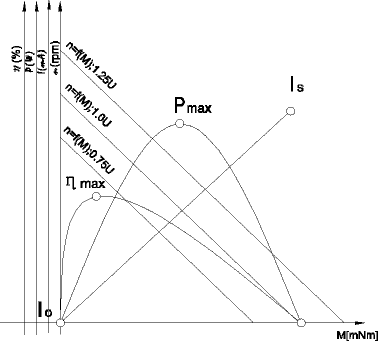You are here: / / / DC Brush Motor

# DC Brush Motor

Views:25     Author:Site Editor     Publish Time: 2018-01-10      Origin:Site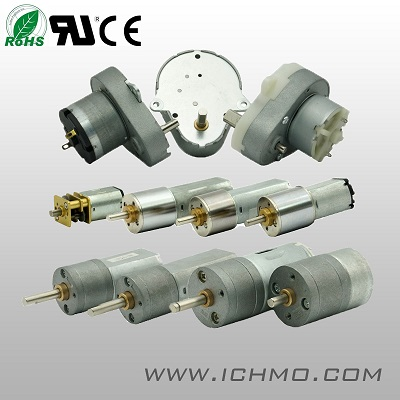DC Brush Motor
Principles of operation
Reference to the chart reveals useful performance information valid for all fulling servomotors.
It shows speed n, current I, output power P and efficiency ¦Ç plotted against torque M for a given supply voltage U. Torque M is a function of the current I and the torque constant k (expressed in Nm/A). The motor develops its maximum torque Ms at stall (n=0), when the current is maximum and determined only by the supply voltage U and the rotor resistance R: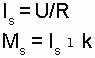With increasing speed, an increasing back EMF E is induced in the armature which tends to reduce the current: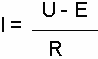The value of E is the product of angular speed ¦Ø (expressed in rad/s) and the torque constant (expressed in V/rad/s=Vs=Nm/A):
E = k¦Ø
Thus, the supply voltage splits into two parts: RI, necessary to establish the current I in the armature, which generates the torque
M, and k¦Ø to overcome the induced voltage, in order to generate the speed¦Ø:
U = RI + k¦Ø
No-load speed no is a function of the supply voltage and is reached when E becomes almost equal to U; no-load current Io is a function of friction torque: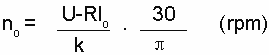Power output P is the product of angular speed¦Ø and torque M (P = M.¦Ø); for a given voltage it reaches its maximum Pmax at half the stall torque Ms, where efficiency is close to 50%. The maximum continuous output power is defined by an hyperbola delimiting the continuous and intermittent operation ranges.
Efficiency¦Çis the mechanical to electrical power ratio (¦Ç= Pm / Pel). Maximum efficiency¦Çmax occurs at relatively high speed. Its value depends upon the ratio of stall torque and friction torque and thus is a function of the supply voltage: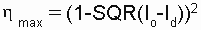The maximum continuous torque depends upon dissipated power (I2R), its maximum value is determined by: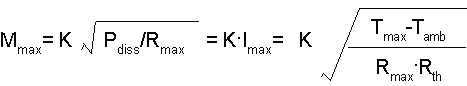Where Tmax is the maximum tolerated armature temperature, Tamb is the ambient temperature, Rmax is the rotor resistance at temperature Tmax and Rth is the total thermal resistance (rotor-body-ambient).
At a given torque M, increasing or decreasing the supply voltage will increase or decrease the speed. The speed-torque function varies proportionally to the supply voltage U.